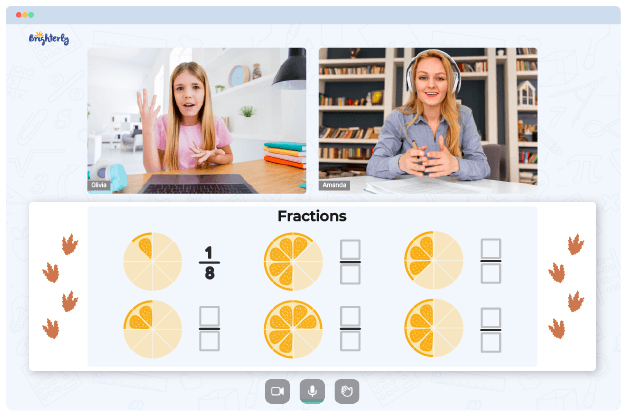# Multiplying Decimals Worksheets

Since multiplication is one of the most challenging concepts kids face, decimal expansion may seem even more complex. However, with a multiply decimals worksheet, kids could learn, practice, and master the multiplication of decimals independently. The variety of questions in multiplying decimals worksheets ensures that kids’ learning experience is enjoyable and exciting.

## Free Multiplying Decimals Worksheets

Multiplying decimals is similar to multiplying whole numbers; decimal points just introduce a new level of complexity to kids. Children begin from the basics of decimal multiplication and then progress to more challenging problems.

Brighterly’s multiplication decimals worksheets come with examples to help kids master the concept of decimal multiplication. These worksheets include solutions to questions in the sheet; therefore, kids will have an insight into how to solve these problems. However, before kids can start solving the questions, they must remember multiplication concepts they have learned before.

## Benefits of Multiplying Decimal Worksheets

With a decimal multiplication worksheet, you can help your kids practice the fundamentals of decimal multiplication and make learning more enjoyable and exciting. Because these math worksheets need lengthy computations, they are set in an engaging and intriguing order.

Math for Kids

Is Your Child Struggling With Math?
1:1 Online Math TutoringThe exciting nature of multiplication with decimals worksheet reinforces the desire of kids to study the topic. Exercises improve logical and critical thinking abilities of kids. The approach used in worksheets coupled with their exciting form guarantee your kids’ success.

Work with decimal multiplication worksheets will give kids the opportunity to solve decimal multiplication problems of various complexity. Furthermore, kids will be required to answer word problems, equations, multiple-choice questions, and problems requiring multiple digit multiplication, among other things.

Brighterly’s multiplying decimals worksheet is designed to aid students in developing a thorough understanding of decimal multiplication. As kids understand this math concept, their ability to reason logically and solve problems improves. Additionally, the flexibility of these worksheets makes it possible for kids to study at their own pace.### Multiply Decimals with Numbers

Decimal Multiplication Worksheet### Multiplying decimals by whole numbers

Multiply Decimals Worksheet### Multiply Decimals with 3 Numbers up to 10

Multiplication Of Decimals Worksheet

## Printable Multiplying Decimals Worksheets

Multiplying decimals worksheets can be an effective tool for students to practice decimal multiplication. These worksheets are available in PDF format and provide questions that gradually increase in difficulty. This is a great way for students to get comfortable with decimal multiplication, as the worksheets start off with basics such as multiplying whole numbers by decimal factors and move on to multiplying decimal numbers by decimal factors. There are also decimal multiplication worksheets that involve mixed operations, allowing students to practice all aspects of decimal multiplication.

With these multiply decimals worksheets, students can gain a better understanding of decimal multiplication and learn how to apply their knowledge in real world situations. By practicing decimal multiplication with these decimal multiplication worksheets, students can become comfortable and confident in multiplying decimal numbers. These decimal multiplication worksheets can be a great way to get students practicing decimal multiplication and help them solidify their understanding of the concept.

Brighterly’s printable multiplying decimals worksheets in PDF format are designed by professional tutors so that students will enjoy working through the materials. The worksheets feature activities, including visual models, practice assignments, and real-world problems. Additionally, there are instructions to guide students as they play.

### More Decimals Worksheets

Struggling with Fractions?• Start studying with an online tutor.

Is your child finding it challenging to grasp fractions lessons? An online tutor could be the answer.